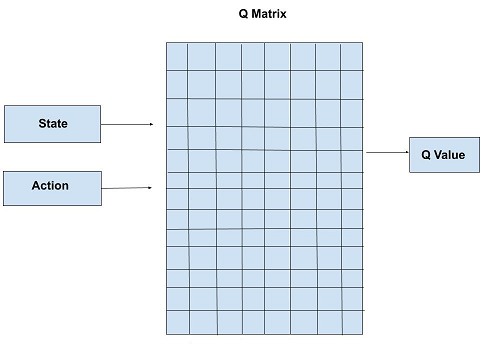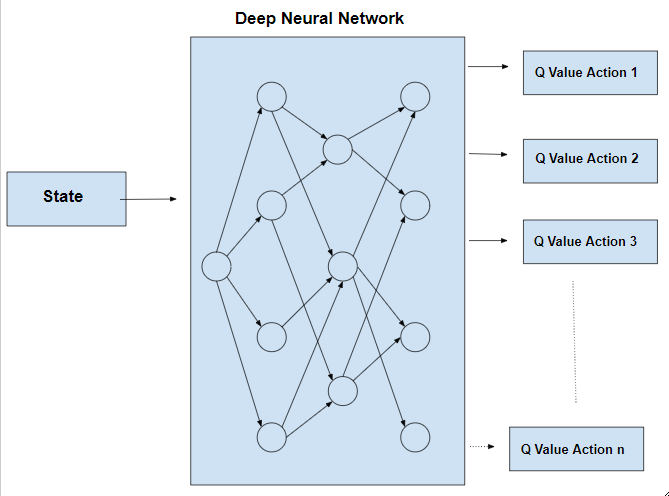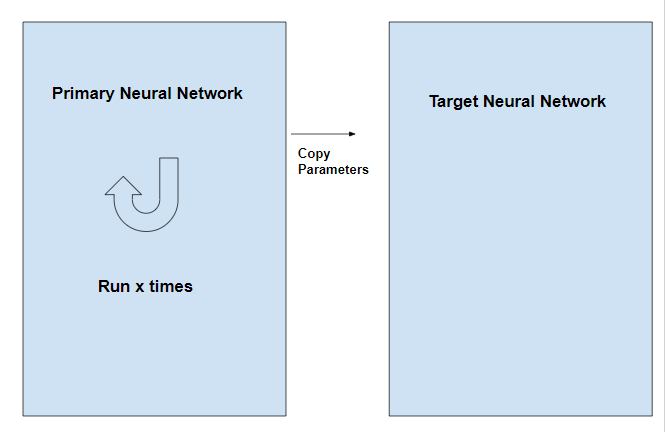# Deep Q-Learning

• Difficulty Level : Easy
• Last Updated : 13 May, 2022

Q-Learning is required as a pre-requisite as it is a process of Q-Learning creates an exact matrix for the working agent which it can “refer to” to maximize its reward in the long run. Although this approach is not wrong in itself, this is only practical for very small environments and quickly loses it’s feasibility when the number of states and actions in the environment increases. The solution for the above problem comes from the realization that the values in the matrix only have relative importance ie the values only have importance with respect to the other values. Thus, this thinking leads us to Deep Q-Learning which uses a deep neural network to approximate the values. This approximation of values does not hurt as long as the relative importance is preserved. The basic working step for Deep Q-Learning is that the initial state is fed into the neural network and it returns the Q-value of all possible actions as an output. The difference between Q-Learning and Deep Q-Learning can be illustrated as follows:Pseudo Code:

Initializefor all pairs (s,a)s = initial statek = 0while(convergence is not achieved){    simulate action a and reach state s'    if(s' is a terminal state)    {        target = R(s,a,s')    }    else    {        target = R(s,a,s') +}s = s'}

Observe that in the equation target = R(s,a,s’) +

*** QuickLaTeX cannot compile formula:

*** Error message:
Error: Nothing to show, formula is empty


, the termis a variable term. Therefore in this process, the target for the neural network is variable unlike other typical Deep Learning processes where the target is stationary. This problem is overcome by having two neural networks instead of one. One neural network is used to adjust the parameters of the network and the other is used for computing the target and which has the same architecture as the first network but has frozen parameters. After an x number of iterations in the primary network, the parameters are copied to the target network.My Personal Notes arrow_drop_up